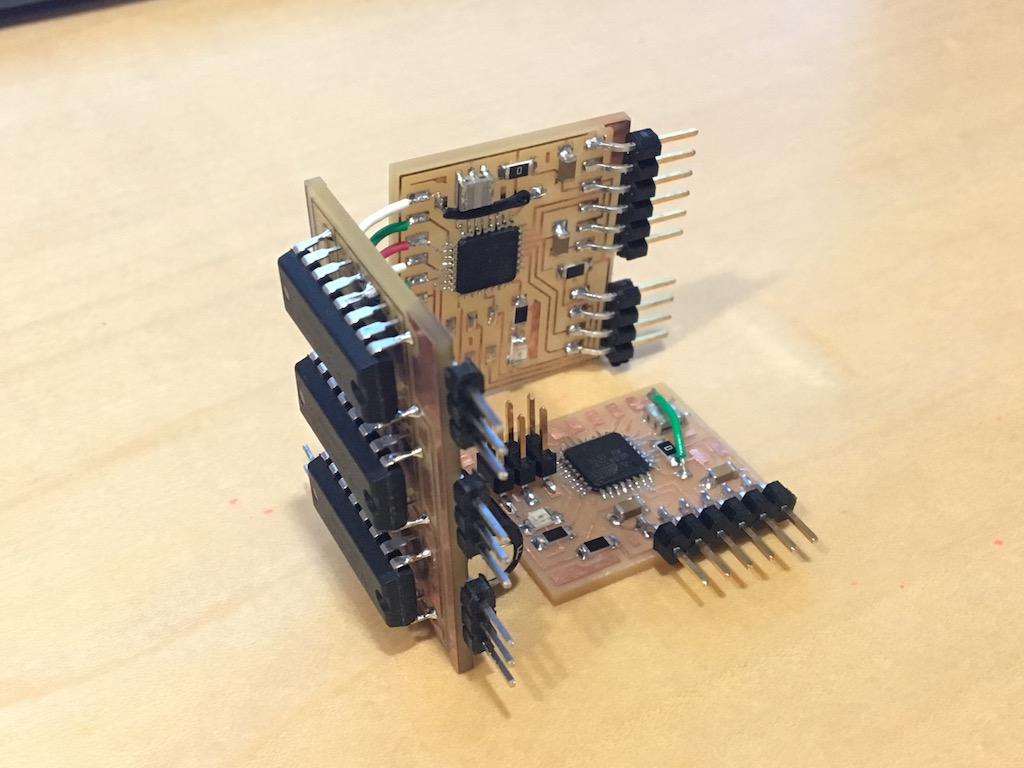Interface programming

### Serial communication between two Atmega328p board.

Because my final project requires three brushless motor control, each require three PWM signals. And Atmega328p can only support 6 channel of PWM, so I need two ATmega board to work together. One of the board read accelarometer and gyroscope data from I2C and need to transmit to the other board. There are multiple ways to do this but I am just using the simple serial communication.

Here are some pictures of the setup. There are two microcontroller chip in the pictures.To transmit one byte of data from one to other is quite simple. All need to do is put the data in the UDR0 register and read it from another board.
However there are two things I need to do is actually not that simple.
1. I need to transmit 16bit data, which in the first thought seems just two 8bit data, but the more tricky thing is how to identify which is the high byte and which is the low byte while data is keep flowing between board. 2. I want to build on interrupt on the receiver instead looping until data comes in. This is essential as I need the microcontroller to keep running the motor.

To solve the first problem, I used 9bit UART instead of 8bit, so the last bit can be used as identifier of the high or low byte of the 16bit data.
On the transmitter side, two functions are created, one to identify as high byte, one as low.

``````
void uart_init(void){
UBRR0L = BRC;
UBRR0H = (BRC >> 8); //Baud rate setting
UCSR0B |= (1 << RXEN0) | (1 << TXEN0); //RxTx enable
UCSR0C = (1 << UCSZ01) | (1 << UCSZ00);
UCSR0B |= (1 << UCSZ02); //9bit serial
}

void uart_setchar_9bit0(char c) {
loop_until_bit_is_set(UCSR0A, UDRE0); /* Wait until data register empty. */
UCSR0B &= ~(1 << TXB80); //9bit zero
UDR0 = c;
}

void uart_setchar_9bit1(char c) {
loop_until_bit_is_set(UCSR0A, UDRE0); /* Wait until data register empty. */
UCSR0B |= (1 << TXB80); //9bit one
UDR0 = c;
} ``````

And for the actually transmitting part. int16_t GyroX is broken into GyroXH and GyroXL and transmitted sequentially.

``````
GyroXH = ((GyroX & 0xFF00)>>8);
uart_setchar_9bit0(GyroXH);
GyroXL = GyroX & 0x00FF;
uart_setchar_9bit1(GyroXL);``````

On the receiver side, interrupt is used.

``````
void uart_init(void){
UBRR0L = BRC;
UBRR0H = (BRC >> 8); //Baud rate setting
UCSR0B |= (1 << RXEN0) | (1 << TXEN0); //RxTx enable
UCSR0B |= (1 << RXCIE0); // rx complete interrupt enable
UCSR0C = (1 << UCSZ01) | (1 << UCSZ00);
UCSR0B |= (1 << UCSZ02); //9bit serial
}``````

The interrupt routine is

``````
ISR(USART_RX_vect){
uint8_t status = UCSR0A;
DataDigit = (UCSR0B >> 1) & 0x01;